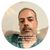# New to Qlik Sense

If you’re new to Qlik Sense, start with this Discussion Board and get up-to-speed quickly.

Announcements
Qlik Cloud Maintenance is scheduled between March 27-30. Visit Qlik Cloud Status page for more details.
cancel
Showing results for
Did you mean:Creator

## Set analysis - Year-to-date

Hi qlik expert.

I have started to use this master calendar; https://community.qlik.com/docs/DOC-8642 , and now I would like to start using the date-function in qlik. I have tried with different set-analysis but with no success.

I would like the sum of values for year-to date, and previous year to the same date (previous year. 04.05.2018 and 04.05.2017)

I have tried using this expression for YTD.

Sum({<Year=, Quarter=, Month=, Week=, Date={‘>=\$(=YearStart(Today()))<=\$(=Today())’}, ElementID= {RR}>} [Vaerdata.Verdi])

But it doesn’t work. Does some have a suggestion? Do I use wrong master calendar script?

Thanks

Cheers
Helge

1 Solution

Accepted SolutionsMVP

May be this

Sum({<

Year = {"\$(=Year(Today()))", "\$(=Year(Today())-1)"},

Quarter,

Month,

Week,

Dato = {"=SetDateYear(Dato, Year(Today())) >= YearStart(Today()) and SetDateYear(Dato, Year(Today())) < Today()"},

ElementID = {RR}>}

[Vaerdata.Verdi])

8 RepliesMVP

Try this

Sum({<Year, Quarter, Month, Week, Date = {">=\$(=YearStart(Today()))<=\$(=Today())"}, ElementID = {RR}>} [Vaerdata.Verdi])

or this

Sum({<Year, Quarter, Month, Week, Date = {">=\$(=Date(YearStart(Today()), 'DateFormatHere'))<=\$(=Date(Today(), 'DateFormatHere'))"}, ElementID = {RR}>} [Vaerdata.Verdi])Creator
Author

by using this expression: sum({<Year= {'2018'}, ElementID = {RR}>} [Vaerdata.Verdi]) I get the correct value, but I would like to learn how to use the date-functions in Qlik.

Do you have any other suggestions of what could be the problem?MVP

You may try two things to troubleshoot-

1. Use the date field in filter pane, if its appearing left aligned means its created as text field, so scriptor expressions need to change to interpret source field as date using date# function.

2. Create test table if used this expression in other charts and check the expression heading, u might see set expression is showing something wrong after Date = {}, inside the curly brackets..MVPCreator
Author

My dates appers to the right in the filter pane. See attached qvf-file.Creator
Author

A copy of the qvf-file is no attached at the my first post.

Cheers HelgeMVP

May be this

Sum({<

Year = {"\$(=Year(Today()))", "\$(=Year(Today())-1)"},

Quarter,

Month,

Week,

Dato = {"=SetDateYear(Dato, Year(Today())) >= YearStart(Today()) and SetDateYear(Dato, Year(Today())) < Today()"},

ElementID = {RR}>}

[Vaerdata.Verdi])Creator
Author

Thanks, Sunny!!

That is exactly what I was searching for!

Cheers HelgeTags
Community Browser